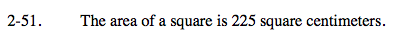### Home > AC > Chapter 14 > Lesson 14.2.1.4 > Problem2-51

2-51.Find the length of one of the sides of the square and use the sides to find the diagonal.

$\sqrt{225}=15\text{ cm}$The Pythagorean Theorem is the following: a2 + b2 = c2

The length of the diagonal is

$15\sqrt{2}$